# Golubev-Privalov theorem

(Redirected from Golubev–Privalov theorem)

Ifis a complex summable function on a closed rectifiable Jordan curvein the complex-plane, then a necessary and sufficient condition for the existence of a function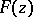, regular in the interior of the domainbounded byand whose angular boundary values coincide withalmost-everywhere on, is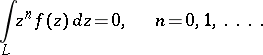(1)

These conditions are known as the Golubev–Privalov conditions. That they are sufficient has been shown by V.V. Golubev ; that they are necessary has been shown by I.I. Privalov . In other words, conditions (1) are necessary and sufficient for the integral of Cauchy–Lebesgue type (cf. Cauchy integral)constructed for the functionand the curve: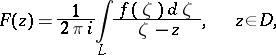to be a Cauchy–Lebesgue integral.

In a more general formulation, letbe a complex Borel measure on. Then the integral of Cauchy–Stieltjes type (cf. Cauchy integral),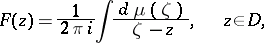is a Cauchy–Stieltjes integral if and only if the generalized Golubev–Privalov conditions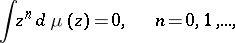(2)

are satisfied.

In other words, conditions (2) are necessary and sufficient for the existence of a regular functioninsuch that its angular boundary values coincide almost-everywhere (with respect to Lebesgue measure) onwith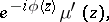where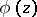is the angle between the positive direction of the abscissa axis and the tangent toat the pointand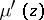is the derivative ofwith respect to Lebesgue measure (arc length) on.

The Golubev–Privalov theorem is of importance in the theory of boundary properties of analytic functions.